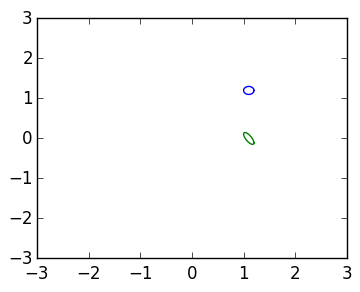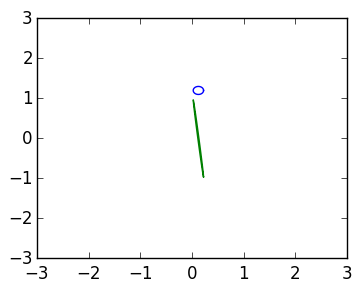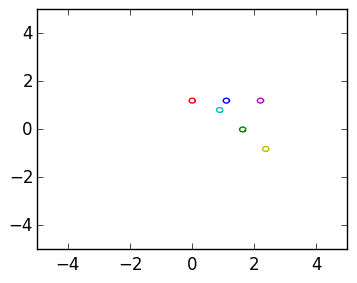# Given's rotations and the QR decomposition¶

In this lecture we introduce Given's rotations as a way to calculate the QR decomposition of a matrix.

We derived an algorithm for calculating the LU Decomposition by applying lower triangular operations on 2 rows at time: thus it came down to the matrix

$$L=\begin{pmatrix} 1 & \cr -{b \over a} & 1 \end{pmatrix}$$

so that

$L\begin{pmatrix} a \cr b \end{pmatrix} = \begin{pmatrix} a \cr 0 \end{pmatrix}$

In :
x=rand(2)
a,b=x;
L=[1    0;
-b/a 1]

L*x

Out:
2-element Array{Float64,1}:
0.398033
1.11022e-16

This had the issue that it degenerated as $a$ becomes small.

As an alternative, we will rotate the vector to introduce a zero. Recall that the angle of a point $(a,b)$ with the origin is given by $\mathrm{atan} {b \over a}$, thus we want to rotate by $\theta = -\mathrm{atan} {b \over a}$:

In :
θ=-atan2(b,a)   # equivalent to  θ=atan(b/a)
Q=[cos(θ) -sin(θ);
sin(θ) cos(θ)]

Q*x

Out:
2-element Array{Float64,1}:
0.959959
5.55112e-17

Unlike lower triangularizations, this works well even for points on the y-axis:

In :
x=[0.,2.]
a,b=x

θ=-atan2(b,a)
Q=[cos(θ) -sin(θ);
sin(θ) cos(θ)]

Q*x

Out:
2-element Array{Float64,1}:
2.0
1.22465e-16

## Effect of error¶

On a computer, we don't know exactly where a point is: every point can have a small $\epsilon$ of error. Thus to understand the robustness of an algorithm, we need to understand what happens to balls of radius $\epsilon$ around where we think of the point. This can be used to demonstrate why rotations are preferred to lower triangular operations.

In the following, we design a function that plots a circle around a point [a,b] of size ε, both before and after a matrix L is applied:

In :
using PyPlot

function plotmat(a,b,ε,L)
t=linspace(0.,2π,100)
x,y=(a+ε*cos(t),b+ε*sin(t))
plot(x,y)

Lx=zeros(length(x))
Ly=zeros(length(x))

for k=1:length(x)
Lx[k],Ly[k]=L*[x[k],y[k]]
end
plot(Lx,Ly)
axis([-3,3,-3,3])
end

Out:
plotmat (generic function with 1 method)

Here we demonstrate that the effect of L is to stretch the circles: our error can be amplified:

In :
a,b=[1.1,1.2]

L=[1    0;
-b/a 1]

ε=0.1

plotmat(a,b,ε,L)Out:
4-element Array{Int64,1}:
-3
3
-3
3

As a becomes small, this error amplification becomes greater: in the following, we go from knowing the true point with accuracy 0.1 to only knowing it with about accuracy 1:

In :
a,b=[0.125,1.2]

L=[1    0;
-b/a 1]

ε=0.1

plotmat(a,b,ε,L)Out:
4-element Array{Int64,1}:
-3
3
-3
3

Rotations perform much better: the circles are only rotated, and are not magnified at all:

In :
a,b=[1.1,1.2]

θ=-atan2(b,a)
Q=[cos(θ) -sin(θ);
sin(θ) cos(θ)]

ε=0.1

plotmat(a,b,ε,Q)
plotmat(0,b,ε,Q)

plotmat(2a,b,ε,Q)
axis([-5,5,-5,5])Out:
4-element Array{Int64,1}:
-5
5
-5
5

# Simpler definition¶

We can construct a simpler expression for Q as $${1 \over \sqrt{a^2 + b^2}}\begin{pmatrix} a & b \cr -b & a \end{pmatrix}$$

In :
Q=[cos(θ) -sin(θ);
sin(θ) cos(θ)]

Out:
2x2 Array{Float64,2}:
0.675725  0.737154
-0.737154  0.675725
In :
[a b; -b a]/sqrt(a^2+b^2)

Out:
2x2 Array{Float64,2}:
0.675725  0.737154
-0.737154  0.675725
In [ ]: